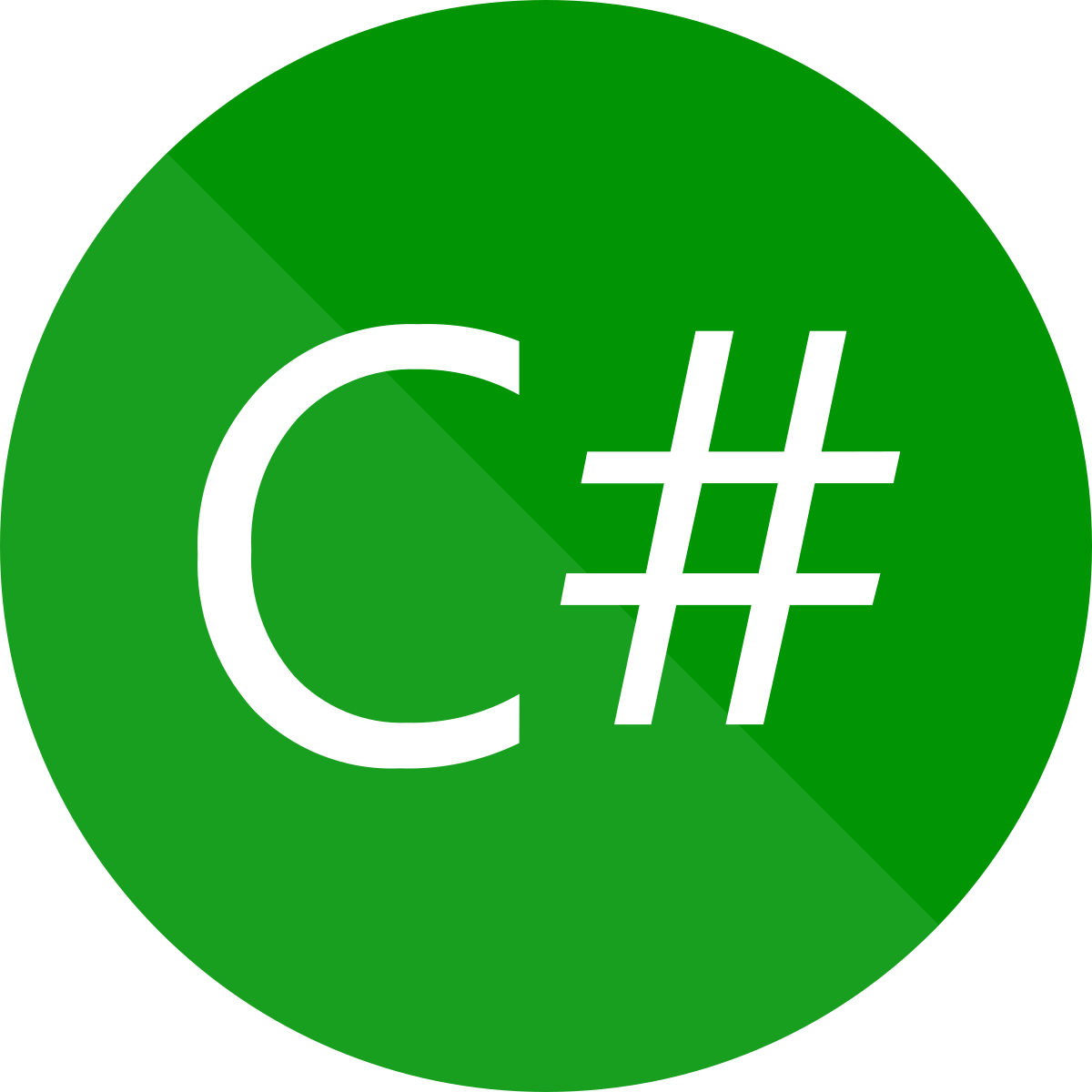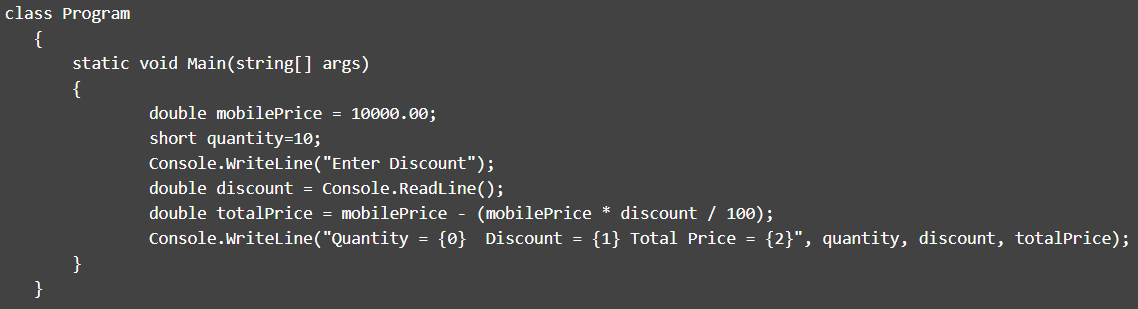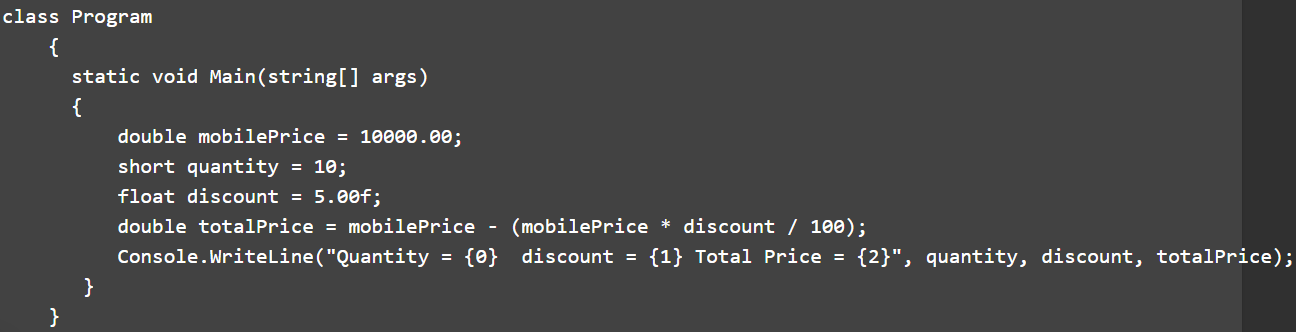0%
26
Created on Byjd

C# ProgrammingC# Programming Quiz 2

This Quiz will test your basic programming knowledge in C#.

1 / 4

Category: C# Programming

Consider the following code snippet. What will be the output if the discount entered is 10.

Choose the output from the options given below:2 / 4

Category: C# Programming

Consider the following code snippet and choose the output from the options given below:3 / 4

Category: C# Programming

Consider the following code snippet and choose the output from the options given below:4 / 4

Category: C# Programming

What is the outcome of the following code snippet?

Note: Array.Rank Property gets the rank (number of dimensions) of the Array.

For example, a one-dimensional array returns 1, a two-dimensional array returns 2, and so on.